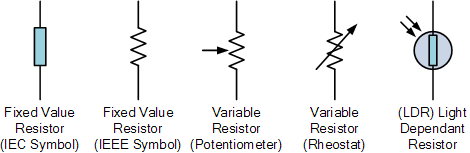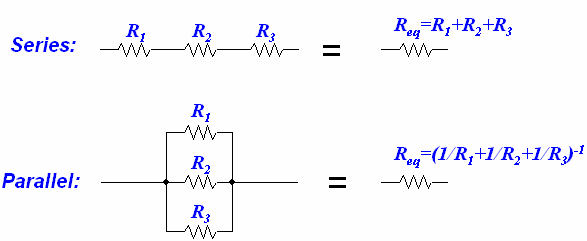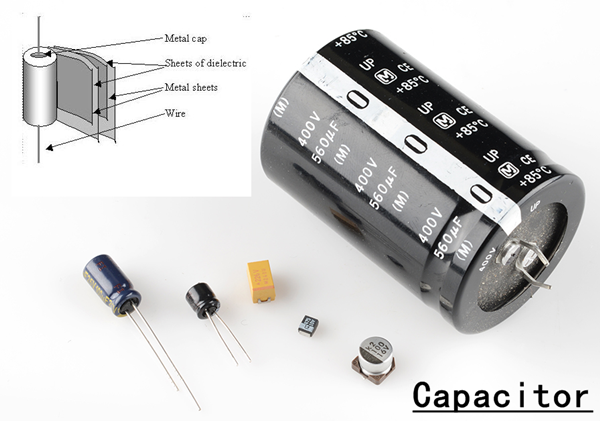# Session 1 (DC Electrics): Introduction

ELECTRIC CHARGE

An Electric Charge is the physical property of matter which causes it to experience a force when placed in an electromagnetic field. There are two types of electric charge which a matter or element can have: Positive & Negative

SI unit of charge is Coulomb (C)

Whenever electrons are made to move through a conductor an electric current is produced. Every material is made up of atoms. Atoms consist of a nucleus and electrons. The electrons possess a negative charge and the nucleus has an equal number of positive charges, this makes an atom electrically neutral. A negative electron is held in its orbit by its attraction to the nucleus. The electrons that are in the outer orbit are not very strongly attached to the nucleus. Hence they may easily fly off or attach themselves to another neighboring atom in the material. Such electrons are called free electrons. An atom that looses an electron is called a positive ion and the one that gains an electron is called a negative ion. Free electrons when made to move in a particular direction through a material, produce an electric current.

Materials that have a large number of free electrons are called conductors and the ones having a very few free electrons are called insulators.

CURRENT

The current flowing through a conductor is measured as the number of electrons that pass any point in the conductor per second.

Electrons flow from negative terminal to positive but direction of current is taken from positive to negative terminal by convention.

SI unit of current is Ampere and symbol is I

Current is measured using a device called Ammeter. An ammeter is connected to a particular circuit so that the current passing through the circuit also passes through the ammeter; hence the reading of the ammeter gives the current flowing through the circuit.

Effects of an Electric Current:

Heating effect: When current flows through a conductor its temperature increase due to its own internal resistance. Used for propeller and windshield anti-icing/de-icing.

Magnetic effect: When current is passed through a coil it creates magnetic effect. Used in motors and generators.

Chemical effect: When an electric current flows through a liquid (like an electrolyte), a chemical change occurs in the liquid as well as the metals immersed in it. Chemical effect of current is used in batteries.

ELECTRO MOTIVE FORCE – EMF (Voltage)

For electric current to flow in a circuit there must be a force behind it. Electro Motive Force is the one that makes the current flow in a conductor. EMF or Potential Difference (i.e. the difference in the electrical potential between a positive and a negative terminal) is measured in units of Voltage. The standardized symbol of voltage is V or E.

If the voltage is increased then the flow of electrons through any point in the circuit also increases and vice-versa. Hence in order to maintain the correct flow it is normal to maintain a constant Voltage in the circuit.

The instrument used for measuring Voltage is known as the Voltmeter. In order to measure the voltage, you have to connect the voltmeter across the two points between which the voltage has to be measured without disconnecting the electric circuit.

SI unit of EMF is Volt

RESISTANCE

Resistance is the obstruction which opposes the flow of current in a conductor.

SI unit of resistance is Ohm.

If a voltage of 1 volt produces a current of 1 ampere, then the material has a resistance of 1 ohm.

Factors affecting resistance:

– Type of material of the conductor (Silver is a better conductor than Copper)

– Length of the conductor (The greater the length more is the resistance)

– Cross-Sectional Area (The thicker a wire, smaller is its resistance)

– Temperature (If the resistance of a conductor increases with the increase in temperature, then the resistor has a Positive Temperature Coefficient [PTC]. If the resistance of a conductor decreases with the increase in temperature, then the resistor has a Negative Temperature Coefficient [NTC]).

Resistors:

We can also adjust the current flowing in a circuit by fitting resistors of known values in the circuit. These resistors can either be fixed or variable resistors.Resistors in Series

If the resistors are connected one after the other in a circuit, then they are said to be connected in Series. Resistor in Series reduces the power consumption as well as the current flow, but if one resistor fails then the rest of the circuit also fails.

We can calculate the Total Circuit Resistance by taking the sum of the individual resistances.

Total Resistance = R1 + R2 + R3 + … + Rn

Resistors in Parallel

If the resistors are connected alongside each other in a circuit, then they are said to be connected in Parallel. Resistors in Parallel ensure that each resistor receives the same voltage and the failure of a single resistor doesn’t affect the whole circuit.

We can calculate the Total Circuit Resistance by using the following formula.

1/Total Resistance = 1/R1 + 1/R2 + 1/R3 + … + 1/RnOHM’s LAW

Ohm’s law states that current flowing through a conductor is directly proportional to potential difference across its end.

Ohms Law is expressed as V = IR

Where V is Voltage, I is current and R is resistance

POWER

Work is said to be done whenever a force produces a movement, Power is said to be the rate at which the work is done. In a circuit work is done by the voltage causing the current to flow through a resistance.

SI Unit of Power is Watts (W)

Formula:

P = Voltage (V) x Amperes (I) or P= I2 x R

CAPACITANCE

Capacitance is the ability of a material to store an electrical charge. Any material that has a large capacitance holds more electric charge at a given voltage, in comparison to the one with low capacitance.

SI Unit of capacitance is Farad [Symbol: F]

1 farad capacitor when charged with 1 coulomb of electrical charge has a potential difference of 1 volt between its plates

Capacitor: A capacitor is a passive two terminal electrical component that is used to store electrical energy temporarily in an electric field.

Capacitors in Series

When the capacitors are connected in series the total capacitance is less than any one of the series capacitors’ individual capacitances.

1/Total Capacitance = 1/C1 + 1/C2 + 1/C3 + … + 1/Cn

Capacitors in Parallel

When the capacitors are connected in parallel the total capacitance is the sum of the individual capacitors’ capacitances.

Total Capacitance = C1 + C2 + C3 + … + Cn

Following images shows inner and outer view of a capacitor for better understanding.KIRCHOFF’s LAWS

Kirchoff’s First Law: Total current flowing into a point on a circuit is equal to the current flowing out of that point.

Kirchoff’s Second Law: If all the voltage drops in a closed electric circuit are added together then their sum is always equal to the voltage applied to that closed circuit.

WHEATSTONE BRIDGE

The arrangement of resistors shown below is known as a Wheatstone Bridge.

Application of Wheatstone Bridge: Wheatstone Bridge is used for comparing an unknown resistance Rx with other resistances of known values. In the given diagram R3 is varied till we get a zero deflection on the ammeter. At this point of time the product of the diagonally opposite resistors are equal to each other as there is no current flow between the point B and C, hence the bridge is said to be balanced.

Thus, we can derive that R1 x RX = R2 x R3

=> RX = R2 x R3 / R1Scroll to Top